Demo problem: Bending of a 3D non-symmetric cantilever beam made of incompressible material

In this tutorial we demonstrate the solution of a 3D solid mechanics problem: the large-amplitude bending deformation of a non-symmetric cantilever beam made of incompressible Mooney-Rivlin material.

Here is an animation of the beam's deformation. In its undeformed configuration, the beam is straight and its cross-section is given by a quarter circle. The beam is loaded by an increasing gravitational body force, acting in the negative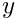-direction, while its left end (at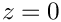) is held fixed. Because of its non-symmetric cross-section, the beam's downward bending deformation is accompanied by a sideways deflection.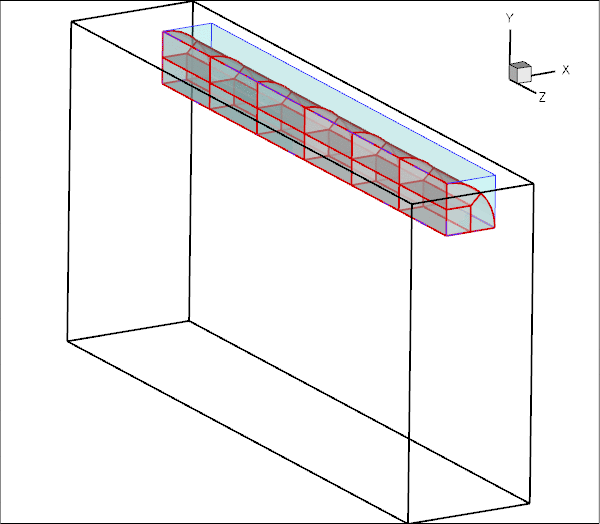Animation of the beam's bending deformation.

Note how the automatic mesh adaptation refines the mesh in the region of strongest bending.

# The mesh

We use multiple inheritance to upgrade the already-existing refineable "quarter tube mesh" to a solid mesh. Following a call to the constructor of the underlying meshes, we set the nodes' Lagrangian coordinates to their current Eulerian positions to make the initial configuration stress-free.

//======================start_mesh=========================================
/// Simple quarter tube mesh upgraded to become a solid mesh
//=========================================================================
template<class ELEMENT>
public virtual RefineableQuarterTubeMesh<ELEMENT>,
public virtual SolidMesh
{
public:
/// Constructor:
RefineableElasticQuarterTubeMesh(GeomObject* wall_pt,
const Vector<double>& xi_lo,
const double& fract_mid,
const Vector<double>& xi_hi,
const unsigned& nlayer,
TimeStepper* time_stepper_pt=
&Mesh::Default_TimeStepper) :
QuarterTubeMesh<ELEMENT>(wall_pt,xi_lo,fract_mid,xi_hi,
nlayer,time_stepper_pt),
RefineableQuarterTubeMesh<ELEMENT>(wall_pt,xi_lo,fract_mid,xi_hi,
nlayer,time_stepper_pt)
{
//Assign the Lagrangian coordinates
set_lagrangian_nodal_coordinates();
}
/// Empty Destructor
};
//////////////////////////////////////////////////////////////////// ////////////////////////////////...
virtual ~RefineableElasticQuarterTubeMesh()
Empty Destructor.
RefineableElasticQuarterTubeMesh(GeomObject *wall_pt, const Vector< double > &xi_lo, const double &fract_mid, const Vector< double > &xi_hi, const unsigned &nlayer, TimeStepper *time_stepper_pt=&Mesh::Default_TimeStepper)
Constructor:

# Global parameters and functions

As usual, we define a namespace, `Global_Physical_Variables`, to define the problem parameters: the length of the cantilever beam,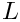, a (pointer to) a strain energy function, the constitutive parameters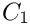and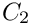for the Mooney-Rivlin strain energy function, and a (pointer to) a constitutive equation. Finally, we define the gravitational body force which acts in the negative-direction.

//=======start_namespace==========================================
/// Global variables
//================================================================
{
/// Length of beam
double L=10.0;
/// Pointer to strain energy function
StrainEnergyFunction* Strain_energy_function_pt=0;
/// First "Mooney Rivlin" coefficient
double C1=1.3;
/// Second "Mooney Rivlin" coefficient
double C2=1.3;
/// Pointer to constitutive law
ConstitutiveLaw* Constitutive_law_pt=0;
/// Non-dim gravity
double Gravity=0.0;
/// Non-dimensional gravity as body force
void gravity(const double& time,
const Vector<double> &xi,
Vector<double> &b)
{
b=0.0;
b=-Gravity;
b=0.0;
}
} //end namespace
//////////////////////////////////////////////////////////////////// ////////////////////////////////...
void gravity(const double &time, const Vector< double > &xi, Vector< double > &b)
Non-dimensional gravity as body force.
ConstitutiveLaw * Constitutive_law_pt
Pointer to constitutive law.
StrainEnergyFunction * Strain_energy_function_pt
Pointer to strain energy function.
double C1
First "Mooney Rivlin" coefficient.
double Gravity
Non-dim gravity.
double C2
Second "Mooney Rivlin" coefficient.

# The driver code

If the code is executed without command line arguments we perform a single simulation. We start by creating the strain energy function and pass it to the constructor of the strain-energy-based constitutive equation. We then build the problem object, using `oomph-lib's` large-displacement Taylor-Hood solid mechanics elements which are based on a continuous-pressure/displacement formulation.

//=======start_of_main==================================================
/// Driver for 3D cantilever beam loaded by gravity
//======================================================================
int main(int argc, char* argv[])
{
// Run main demo code if no command line arguments are specified
if (argc==1)
{
// Create incompressible Mooney Rivlin strain energy function
new MooneyRivlin(&Global_Physical_Variables::C1,
// Define a constitutive law (based on strain energy function)
new IsotropicStrainEnergyFunctionConstitutiveLaw(
//Set up the problem with continous pressure/displacement
Problem class for the 3D cantilever "beam" structure.
int main(int argc, char *argv[])
Driver for 3D cantilever beam loaded by gravity.

We document the initial configuration before starting a parameter study in which the magnitude of the gravitational body force is increased in small steps:

// Doc solution
problem.doc_solution();
// Initial values for parameter values
//Parameter incrementation
unsigned nstep=10;
double g_increment=5.0e-4;
for(unsigned i=0;i<nstep;i++)
{
// Solve the problem with Newton's method, allowing
// Doc solution
problem.doc_solution();
}
} // end main demo code
void doc_solution()
Doc the solution.

If the code is executed with a non-zero number of command line arguments, it performs a large number of additional self tests that we will not discuss here. See the driver code three_d_cantilever.cc for details.

# The problem class

The problem class contains the usual member functions. No action is required before the mesh adaptation; we overload the function `Problem::actions_after_adapt()` to pin the redundant solid pressure degrees of freedom afterwards.

//=============begin_problem============================================
/// Problem class for the 3D cantilever "beam" structure.
//======================================================================
template<class ELEMENT>
class CantileverProblem : public Problem
{
public:
/// Constructor:
/// Update function (empty)
/// Update function (empty)
{
// Pin the redundant solid pressures (if any)
PVDEquationsBase<3>::pin_redundant_nodal_solid_pressures(
mesh_pt()->element_pt());
}
/// Doc the solution
void doc_solution();
void actions_before_newton_solve()
Update function (empty)
void actions_after_newton_solve()
Update function (empty)
CantileverProblem()
Constructor:
RefineableElasticQuarterTubeMesh< ELEMENT > * mesh_pt()
Access function for the mesh.

We overload the `Problem::mesh_pt()` function to return a pointer to the specific mesh used in this problem:

/// Access function for the mesh
{
Problem::mesh_pt());
}

The private member data stores a `DocInfo` object in which we will store the name of the output directory.

private:
/// DocInfo object for output
DocInfo Doc_info;
};

# The problem constructor

We start by creating the `GeomObject` that defines the curvilinear boundary of the beam: a circular cylinder of unit radius.

//===========start_of_constructor=======================================
/// Constructor:
//======================================================================
template<class ELEMENT>
{
// Create geometric object that defines curvilinear boundary of
// beam: Elliptical tube with half axes = radius = 1.0
// Bounding Lagrangian coordinates
Vector<double> xi_lo(2);
xi_lo=0.0;
xi_lo=0.0;
Vector<double> xi_hi(2);
xi_hi=2.0*atan(1.0);

We build the mesh, using six axial layers of elements, before creating an error estimator and specifying the error targets for the adaptive mesh refinement.

// # of layers
unsigned nlayer=6;
//Radial divider is located half-way along the circumference
double frac_mid=0.5;
//Now create the mesh
(wall_pt,xi_lo,frac_mid,xi_hi,nlayer);
// Set error estimator
mesh_pt())->spatial_error_estimator_pt()=new Z2ErrorEstimator;
// Error targets for adaptive refinement
mesh_pt()->max_permitted_error()=0.05;
mesh_pt()->min_permitted_error()=0.005;

We complete the build of the elements by specifying the constitutive equation and the body force. We check that the element is based on a pressure/displacement formulation, and, if so, select an incompressible formulation. (This check is only required because the self-tests not shown here also include cases in which the problem is solved using a displacement-based formulation with compressible elasticity; see also the section How to enforce incompressibility below).

// Complete build of elements
unsigned n_element=mesh_pt()->nelement();
for(unsigned i=0;i<n_element;i++)
{
// Cast to a solid element
ELEMENT *el_pt = dynamic_cast<ELEMENT*>(mesh_pt()->element_pt(i));
// Set the constitutive law
el_pt->constitutive_law_pt() =
// Set the body force
el_pt->body_force_fct_pt() = Global_Physical_Variables::gravity;
// Material is incompressble: Use incompressible displacement/pressure
// formulation (if the element is pressure based, that is!)
PVDEquationsWithPressure<3>* cast_el_pt =
dynamic_cast<PVDEquationsWithPressure<3>*>(mesh_pt()->element_pt(i));
if (cast_el_pt!=0)
{
cast_el_pt->set_incompressible();
}
} // done build of elements

We fix the position of all nodes at the left end of the beam (on boundary 0) and pin any redundant solid pressures.

// Pin the left boundary (boundary 0) in all directions
unsigned b=0;
unsigned n_side = mesh_pt()->nboundary_node(b);
//Loop over the nodes
for(unsigned i=0;i<n_side;i++)
{
mesh_pt()->boundary_node_pt(b,i)->pin_position(0);
mesh_pt()->boundary_node_pt(b,i)->pin_position(1);
mesh_pt()->boundary_node_pt(b,i)->pin_position(2);
}
// Pin the redundant solid pressures (if any)
PVDEquationsBase<3>::pin_redundant_nodal_solid_pressures(
mesh_pt()->element_pt());

Finally, we assign the equation numbers and define the output directory.

//Assign equation numbers
assign_eqn_numbers();
// Prepare output directory
Doc_info.set_directory("RESLT");
} //end of constructor

# Post-processing

The post-processing function `doc_solution()` simply outputs the shape of the deformed beam.

//==============start_doc===========================================
/// Doc the solution
//==================================================================
template<class ELEMENT>
{
ofstream some_file;
char filename;
// Number of plot points
unsigned n_plot = 5;
// Output shape of deformed body
sprintf(filename,"%s/soln%i.dat",Doc_info.directory().c_str(),
Doc_info.number());
some_file.open(filename);
mesh_pt()->output(some_file,n_plot);
some_file.close();
// Increment label for output files
Doc_info.number()++;
} //end doc

## How to enforce incompressibility

We stress that the imposition of incompressibility must be requested explicitly via the element's member function `incompressible()`. Mathematically, incompressibility is enforced via a Lagrange multiplier which manifests itself physically as the pressure. Incompressibility can therefore only be enforced for elements that employ the pressure-displacement formulation of the principle of virtual displacements. This is why we the `Problem` constructor checked if the element is derived from the `PVDEquationsWithPressure` class before setting the element's `incompressible` flag to `true`.

As usual, `oomph-lib` provides self-tests that assess if the enforcement incompressibility (or the lack thereof) is consistent:

• The compiler will not allow the user to enforce incompressibility on elements that are based on the displacement form of the principle of virtual displacements.

• Certain constitutive laws, such as the Mooney-Rivlin law used in the present example require an incompressible formulation. If `oomph-lib` is compiled with the `PARANOID` flag, an error is thrown if such a constitutive law is used by an element for which incompressibility has not been requested.

Recall that the default setting is not to enforce incompressibility!

If the library is compiled without the `PARANOID` flag no warning will be issued but the results will be "wrong" at least in the sense that the material does not behave like an incompressible Mooney-Rivlin solid. In fact, it is likely that the Newton solver will diverge. Anyway, as we keep saying, without the `PARANOID` flag, you're on your own!

• Some constitutive laws can be used for compressible and incompressible behaviour. In this case it is important to set the `incompressible()` flag to the correct value. This issue is discussed in more detail in another tutorial.

You should experiment with different combinations of constitutive laws and element types to familiarise yourself with these.